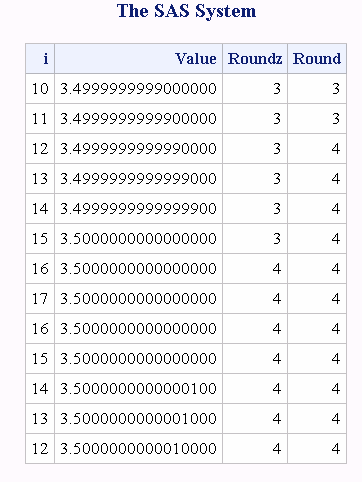# ROUNDZ Function

Rounds the first argument to the nearest multiple of the second argument, using zero fuzzing.

 Category: Truncation

## Syntax

ROUNDZ (argument )

### Required Argument

#### argument

is a numeric constant, variable, or expression to be rounded.

### Optional Argument

#### rounding-unit

is a positive, numeric constant, variable, or expression that specifies the rounding unit.

## Details

The ROUNDZ function rounds the first argument to the nearest multiple of the second argument. If you omit the second argument, ROUNDZ uses a default value of 1 for rounding-unit.

## Comparisons

The ROUND, ROUNDE, and ROUNDZ functions are similar with four exceptions:
• ROUND returns the multiple with the larger absolute value when the first argument is approximately halfway between the two nearest multiples of the second argument.
• ROUNDE returns an even multiple when the first argument is approximately halfway between the two nearest multiples of the second argument.
• ROUNDZ returns an even multiple when the first argument is exactly halfway between the two nearest multiples of the second argument.
• When the rounding unit is less than one and not the reciprocal of an integer, the result that is returned by ROUNDZ might not agree exactly with the result from decimal arithmetic. ROUND and ROUNDE perform extra computations, called fuzzing, to try to make the result agree with decimal arithmetic in the most common situations. ROUNDZ does not fuzz the result.

## Examples

### Example 1: Comparing Results from the ROUNDZ and ROUND Functions

The following example compares the results that are returned by the ROUNDZ and the ROUND function.
```data test;
do i=10 to 17;
Value=3.5 - 10**(-i);
Roundz=roundz(value);
Round=round(value);
output;
end;
do i=16 to 12 by -1;
value=3.5 + 10**(-i);
roundz=roundz(value);
round=round(value);
output;
end;
run;
proc print data=test noobs;
format value 19.16;
run;```
Results That Are Returned by the ROUNDZ and ROUND Functions### Example 2: Sample Output from the ROUNDZ Function

These examples show the results that are returned by ROUNDZ.
Results Using ROUNDZ
SAS Statement
Result
```var1=223.456;
x=roundz(var1,1);
put x 9.5;```
```
223.00000```
```var2=223.456;
x=roundz(var2,.01);
put x 9.5;```
```
223.46000```
```x=roundz(223.456,100);
put x 9.5;```
```
200.00000```
```x=roundz(223.456);
put x 9.5;```
```
223.00000```
```x=roundz(223.456,.3);
put x 9.5;```
```
223.50000```